## 4.8Numerical framework

The ﬁxed value and ﬁxed gradient conditions described in Sec. 4.2 can be combined to form a general numerical framework for boundary conditions.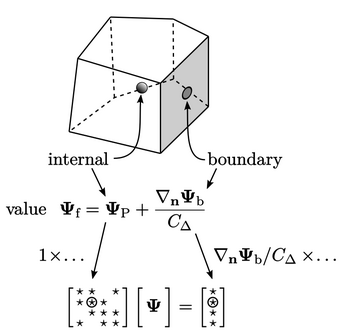The contributions from the boundary conditions to the matrix equation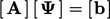, by discretisation of advection and Laplacian terms, can be generalised as:

• internal” contributions to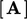, from terms including the cell value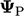;
• boundary” contributions to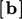, from terms without.

The example above shows Eq. (4.2 ) for the face value, required at a boundary for advection discretisation, in the case of a ﬁxed gradient boundary condition. The internal “factor” on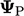is 1, which is multiplied by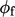for the contribution to the respective diagonal coeﬃcient in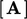, as in the example in Sec. 3.24 .

The boundary factor is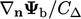, which is similarly multiplied by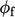for the contribution to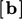.

For the ﬁxed value condition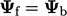with advection, the boundary factor is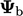and an internal factor is 0.

Laplacian discretisation requires the surface normal gradient on the faces. A ﬁxed gradient condition delivers an equivalent boundary factor of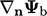to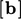and an internal factor of 0.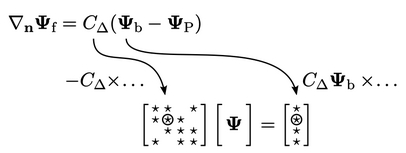With a ﬁxed value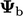, the face normal gradient Eq. (4.3 ) gives an internal factor of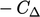and a boundary factor of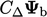. Both are multiplied by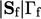in their contributions to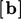and the diagonal coeﬃcient of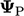in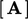, as shown in the Laplacian discretisation in Sec. 3.24 .

The table below summarises: the “value” internal and boundary factors, contributing to the respective matrix coeﬃcients with advection discretisation; and equivalent “gradient” factors relating to Laplacian discretisation. This provides a framework which can be extended to more complex conditions.

 term factor ﬁxed value ﬁxed gradient advection value internal 0 1 advection value boundary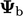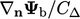Laplacian gradient internal0 Laplacian gradient boundary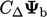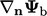Notes on CFD: General Principles - 4.8 Numerical framework